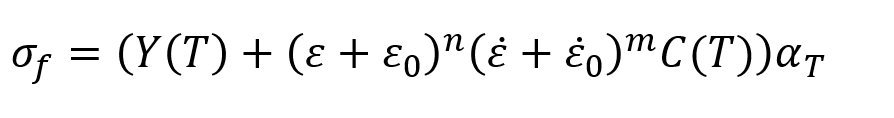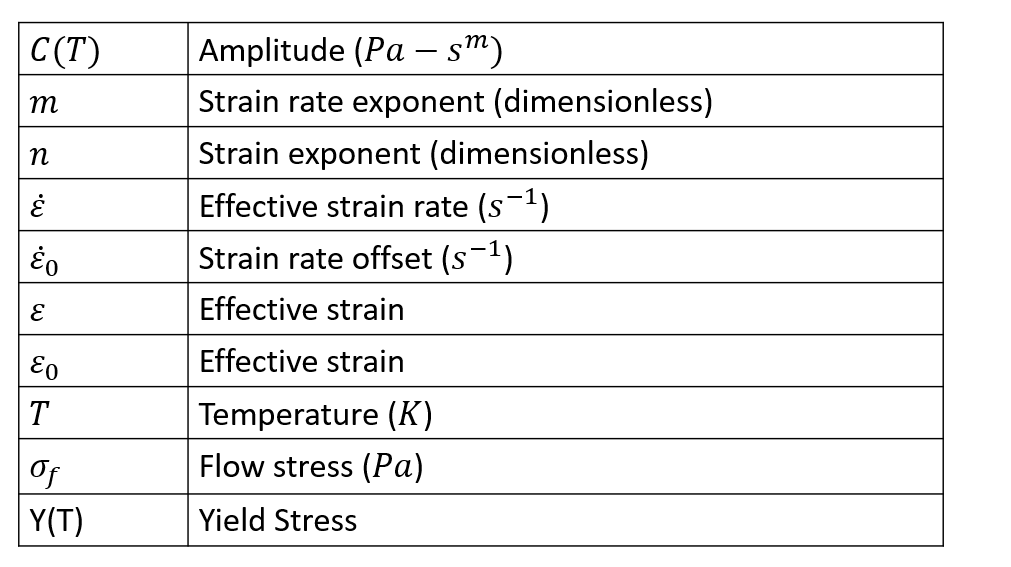# Metal Forming Power Law

The generalized power law used in HyperXtrude is given by the following expression.Depending on the choice for the variable Temperature Dependence, four different variants of the PowerLaw model can be obtained. Valid entries for the variable TemperatureDependence can be None, Coefficients, Exp(Q/RT), or Exp(-Beta(DeltaT)). Syntax for PowerLaw model with temperature dependence None is given below.

 Workpiece Aluminum { ConstitutveModel = "PowerLaw" Density = ρ SpecificHeat = Cp (T) COnductivity = K (T) CoeffOfThermalExpansion = βT VolumetricHeatSource = Qvol Amplitude = C ConstantY = Y StrainRateHardeningExponent = m StainHardeningExponent = n Reference Temperature = T0 Solidus Temperature = Ts LiquidusTemeprature = T1 TemperatureDependence = "None" StrainOffset = ε1 StrainRateOffset = ε2 YoungModulus = E PoissonRatio = ν }

## Valid Data for Temperature Dependence

Field Description
None Even for the case where there is no temperature dependence, a reference temperature should be specified. In this case, the properties are computed at this temperature.
Coefficients With this option, the parameters Amplitude and ConstantY can depend on temperature and you can specify them using a TABLE or FUNCTION. The only difference between the Coefficients and None is that properties are constant in the case on None.
Exp(Q/RT) In this case, in addition to ReferenceTemperature (T0), two other parameters, ActivationEnergy (Q) and UniversalGasConstant (R), are needed by the model. The following two lines should be added to the syntax.
Exp(-Beta (DeltaT))

In this case, in addition to ReferenceTemperature (T), parameters, Beta (beta) is needed by the model. The following line should be added to the original syntax.

Beta = β